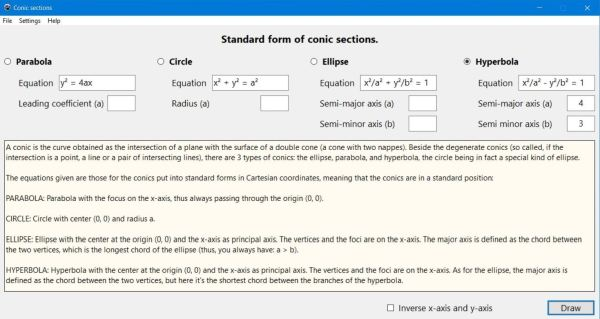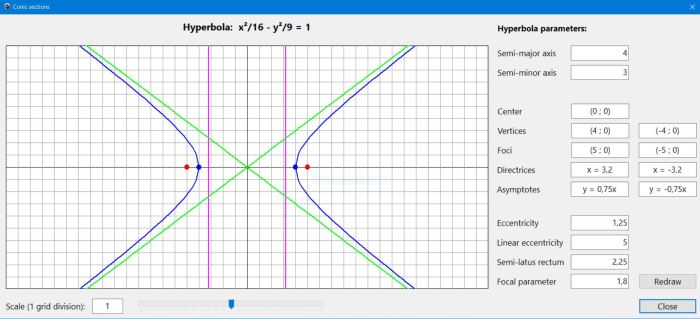# Computing: Free Pascal Programming

 Programming Home Home Contact

## Conic sections (Conics).

Description: Geometry application, dealing with the conic sections (parabola, ellipse, circle, hyperbola) in their standard form. The user chooses the conic type and enters the conic's equation arguments (leading coefficient for the parabola, radius for the circle, semi-major and semi-minor axis for the ellipse and the hyperbola) and the application calculates the most relevant values of the conic (vertices, foci, directrices, the hyperbola's asymptotes, eccentricity, linear eccentricity, semi-latus rectun, focal parameter) and, of course, draws the conic's curve.
A trackbar may be used to reduce or enlarge the drawing (selected values between 50:1 and 1:50). This allows proper drawings for a large range of equation arguments (if these are to small or to big, nothing will be drawn or the graph will not be readable...).

Possible extensions: The only parameters actually drawn on the graph are the center, always (0, 0) in standard form, the vertices and foci, the directrices and, for the hyperbola, the asymptotes. Drawing all relevant parameters should not be to difficult to implement... A more complex extension could be to consider a more general form of the conic, letting the user choose a given center.

Free Pascal features: Showing/hiding controls as needed; changing label captions during run-time. Drawing mathematical curves, using bitmap canvas.

Screenshots: